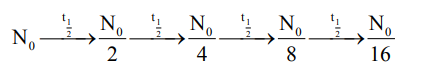# A radioactive substance decays toQuestion:

A radioactive substance decays to $\left(\frac{1}{16}\right)^{\text {th }}$ of its initial activity in 80 days. The half life of the radioactive substance expressed in days is________.

Solution:$4 \times t_{1 / 2}=80$

$t_{1 / 2}=20$ days